• 用C++求图的最小生成树、单元点最短路径、两点之间最短路径
• 展开全部Dijkstra(迪杰斯特拉)算法是典型的最短路径路由算法，用于计算一个节点到其他所有节e68a8462616964757a686964616f31333361316131最短路径。主要特点是以起始为中心向外层层扩展，直到扩展到终点为止。...
展开全部Dijkstra(迪杰斯特拉)算法是典型的最短路径路由算法，用于计算一个节点到其他所有节e68a8462616964757a686964616f31333361316131点的最短路径。主要特点是以起始点为中心向外层层扩展，直到扩展到终点为止。Dijkstra一般的表述通常有两种方式，一种用永久和临时标号方式，一种是用OPEN, CLOSE表方式用OPEN,CLOSE表的方式，其采用的是贪心法的算法策略，大概过程如下：1.声明两个集合，open和close，open用于存储未遍历的节点，close用来存储已遍历的节点2.初始阶段，将初始节点放入close，其他所有节点放入open3.以初始节点为中心向外一层层遍历，获取离指定节点最近的子节点放入close并从新计算路径，直至close包含所有子节点代码实例如下：Node对象用于封装节点信息，包括名字和子节点[java] view plain copypublic class Node {private String name;private Map child=new HashMap();public Node(String name){this.name=name;}public String getName() {return name;}public void setName(String name) {this.name = name;}public Map getChild() {return child;}public void setChild(Map child) {this.child = child;}}MapBuilder用于初始化数据源，返回图的起始节点[java] view plain copypublic class MapBuilder {public Node build(Set open, Set close){Node nodeA=new Node("A");Node nodeB=new Node("B");Node nodeC=new Node("C");Node nodeD=new Node("D");Node nodeE=new Node("E");Node nodeF=new Node("F");Node nodeG=new Node("G");Node nodeH=new Node("H");nodeA.getChild().put(nodeB, 1);nodeA.getChild().put(nodeC, 1);nodeA.getChild().put(nodeD, 4);nodeA.getChild().put(nodeG, 5);nodeA.getChild().put(nodeF, 2);nodeB.getChild().put(nodeA, 1);nodeB.getChild().put(nodeF, 2);nodeB.getChild().put(nodeH, 4);nodeC.getChild().put(nodeA, 1);nodeC.getChild().put(nodeG, 3);nodeD.getChild().put(nodeA, 4);nodeD.getChild().put(nodeE, 1);nodeE.getChild().put(nodeD, 1);nodeE.getChild().put(nodeF, 1);nodeF.getChild().put(nodeE, 1);nodeF.getChild().put(nodeB, 2);nodeF.getChild().put(nodeA, 2);nodeG.getChild().put(nodeC, 3);nodeG.getChild().put(nodeA, 5);nodeG.getChild().put(nodeH, 1);nodeH.getChild().put(nodeB, 4);nodeH.getChild().put(nodeG, 1);open.add(nodeB);open.add(nodeC);open.add(nodeD);open.add(nodeE);open.add(nodeF);open.add(nodeG);open.add(nodeH);close.add(nodeA);return nodeA;}}图的结构如下图所示：Dijkstra对象用于计算起始节点到所有其他节点的最短路径[java] view plain copypublic class Dijkstra {Set open=new HashSet();Set close=new HashSet();Map path=new HashMap();//封装路径距离Map pathInfo=new HashMap();//封装路径信息public Node init(){//初始路径,因没有A->E这条路径,所以path(E)设置为Integer.MAX_VALUEpath.put("B", 1);pathInfo.put("B", "A->B");path.put("C", 1);pathInfo.put("C", "A->C");path.put("D", 4);pathInfo.put("D", "A->D");path.put("E", Integer.MAX_VALUE);pathInfo.put("E", "A");path.put("F", 2);pathInfo.put("F", "A->F");path.put("G", 5);pathInfo.put("G", "A->G");path.put("H", Integer.MAX_VALUE);pathInfo.put("H", "A");//将初始节点放入close,其他节点放入openNode start=new MapBuilder().build(open,close);return start;}public void computePath(Node start){Node nearest=getShortestPath(start);//取距离start节点最近的子节点,放入closeif(nearest==null){return;}close.add(nearest);open.remove(nearest);Map childs=nearest.getChild();for(Node child:childs.keySet()){if(open.contains(child)){//如果子节点在open中Integer newCompute=path.get(nearest.getName())+childs.get(child);if(path.get(child.getName())>newCompute){//之前设置的距离大于新计算出来的距离path.put(child.getName(), newCompute);pathInfo.put(child.getName(), pathInfo.get(nearest.getName())+"->"+child.getName());}}}computePath(start);//重复执行自己,确保所有子节点被遍历computePath(nearest);//向外一层层递归,直至所有顶点被遍历}public void printPathInfo(){Set> pathInfos=pathInfo.entrySet();for(Map.Entry pathInfo:pathInfos){System.out.println(pathInfo.getKey()+":"+pathInfo.getValue());}}/*** 获取与node最近的子节点*/private Node getShortestPath(Node node){Node res=null;int minDis=Integer.MAX_VALUE;Map childs=node.getChild();for(Node child:childs.keySet()){if(open.contains(child)){int distance=childs.get(child);if(distanceminDis=distance;res=child;}}}return res;}}Main用于测试Dijkstra对象[java] view plain copypublic class Main {public static void main(String[] args) {Dijkstra test=new Dijkstra();Node start=test.init();test.computePath(start);test.printPathInfo();}}本回答由提问者推荐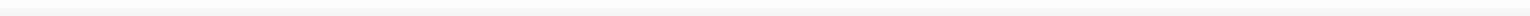已赞过已踩过<你对这个回答的评价是？评论收起
展开全文• 探路者 使用A *寻路找到两点之间最短路径的程序Python
• 这是使用BFS从(0,0)到(0，m-1)的矩阵中的最短路径的python实现 . 您可以将其更改为适合变量 .n,m,k1,k2=[int(i) for i in input().split()]arr=[[int(j) for j in input().split()] for i in range(n)]x=[[-1 for ...
这是使用BFS从(0,0)到(0，m-1)的矩阵中的最短路径的python实现 . 您可以将其更改为适合变量点 .n,m,k1,k2=[int(i) for i in input().split()]arr=[[int(j) for j in input().split()] for i in range(n)]x=[[-1 for i in range(m)] for j in range(n)]x=0vis={}q=[(0,0)]while len(q)!=0:curr=qrem=q.pop(0)vis[curr]=Truer=currc=currif r-1>=0 and arr[r-1][c]==0:if vis.get((r-1,c),-1)==-1 or vis[(r-1,c)]!=True:q.append((r-1,c))x[r-1][c]=x[r][c]+1if r+1if vis.get((r+1,c),-1)==-1 or vis[(r+1,c)]!=True:q.append((r+1,c))x[r+1][c]=x[r][c]+1if c-1>=0 and arr[r][c-1]==0:if vis.get((r,c-1),-1)==-1 or vis[(r,c-1)]!=True:q.append((r,c-1))x[r][c-1]=x[r][c]+1if c+1if vis.get((r,c+1),-1)==-1 or vis[(r,c+1)]!=True:q.append((r,c+1))x[r][c+1]=x[r][c]+1#for i in x:#print(i)ans=x[m-1]if ans==-1:print(-1)else:print(ans)输入矩阵应包含0 's and 1' s .  0表示可能的移动 .n是行数 .m是列数 .arr是给定的矩阵 .x是距离(0,0)的距离矩阵 .vis是一个字典，如果访问该节点则给出一个布尔值 .输出-1表示没有这样的路径可能 .
展开全文• 由于这是一个课程项目，时间比较急，而且自己不熟悉A*算法，所以参考网上的Dijkstra算法(http://blog.csdn.net/javaman_chen/article/details/8254309)的代码来实现了地图上任意两点最短路径的查询。但该demo存在...
地图上实现最短路径的查询，据我了解的，一般用Dijkstra算法和A*算法来实现。由于这是一个课程项目，时间比较急，而且自己不熟悉A*算法，所以参考网上的Dijkstra算法(http://blog.csdn.net/javaman_chen/article/details/8254309)的代码来实现了地图上任意两点的最短路径的查询。但该demo存在一个很严重的错误，缺了两行非常关键的代码……首先，来了解下Dijkstra算法：无向图的最短路径求解算法之——Dijkstra算法http://sbp810050504.blog.51cto.com/2799422/690803 。由此可以看出，Dijkstra算法的效率是很低的，它遍历的点很多，要以起始点为中心向外层层扩展，直到扩展到终点为止，所以数据量很少时不适合Dijkstra算法。处理该算法时，要特别注意在由一个点找到相邻该点最近点的时候，记得要将相邻的点的距离更新。Dijkstra一般的表述通常有两种方式，一种用永久和临时标号方式，一种是用OPEN, CLOSE表的方式，这里是采用第二种方式，也就是采用的贪心法的算法策略，大概过程如下：1.定义两个集合:open和close，open用于存储未遍历的节点，close用来存储已遍历的节点;2.初始阶段，将初始节点放入close，其他所有节点放入open;3.以初始节点为中心向外一层层遍历，获取离指定节点最近的子节点放入close并重新更新相邻点的距离，直至close包含所有子节点；此方法由一个点遍历了其他所有点，所以可以知道该点到其他所有点的距离，时间复杂度很高。那个demo是一个一个数据初始化的，我将它改为用数组存储，然后用for循环来初始化，也就是将它封装成我需要的数据接口，并没有很大的优化。算法核心只有两个函数：/*** 获取与node最近的子节点*/privateNode getShortestPath(Node node) {Node res= null;int minDis =Integer.MAX_VALUE;Map childs =node.getChild();//循环比较，找出离node最近的子节点for(Node child : childs.keySet()) {if(open.contains(child)) {int distance =childs.get(child);if (distance minDis=distance;res=child;}}}returnres;}View Codepublic voidcomputePath(Node start) {Node nearest= getShortestPath(start);//取距离start节点最近的子节点,放入closeif (nearest == null) {return;}close.add(nearest);open.remove(nearest);Map childs =nearest.getChild();for(Node child : childs.keySet()) {if (open.contains(child)) {//如果子节点在open中Integer newCompute = path.get(nearest.getName()) +childs.get(child);if (path.get(child.getName()) > newCompute) {//之前设置的距离大于新计算出来的距离path.put(child.getName(), newCompute);start.getChild().put(child, newCompute);close.add(start);pathInfo.put(child.getName(), pathInfo.get(nearest.getName())+ "-" +child.getName());}}}computePath(start);//重复执行自己,确保所有子节点被遍历computePath(nearest);//向外一层层递归,直至所有顶点被遍历}View Code那个demo漏了两行代码，就是找到最近的路径后，没有更新相邻点的距离start.getChild().put(child, newCompute);close.add(start);还有很多东西没优化，欢迎指出，一起学习！
展开全文• 两点之间最短路径的计算-floyd算法 Floyd算法的核心内容 算法过程图解 伪代码 完整代码 void floyd() { for (int k = 0; k < n; ++k) { for (int i = 0; i < n; ++i) { for (int j = 0; j < n; +...
两点之间最短路径的计算-floyd算法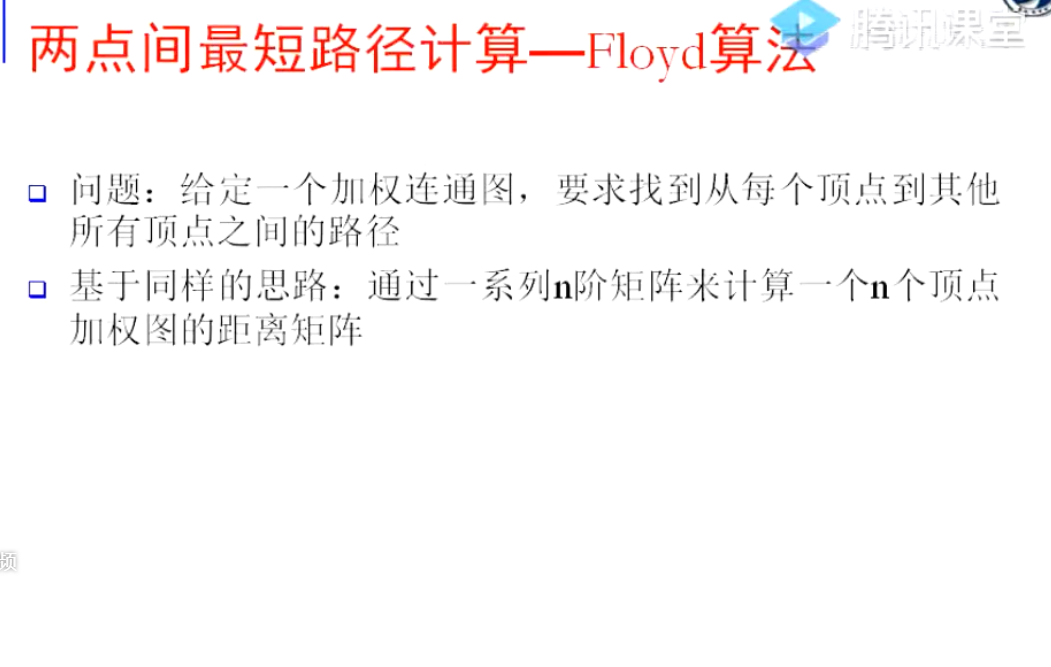Floyd算法的核心内容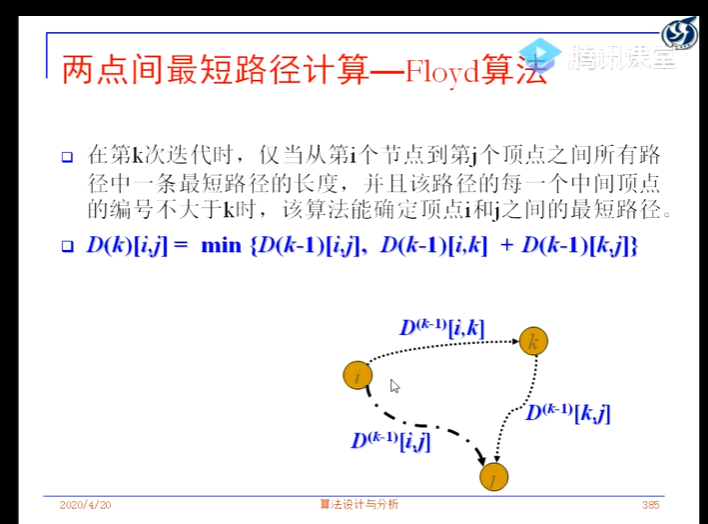算法过程图解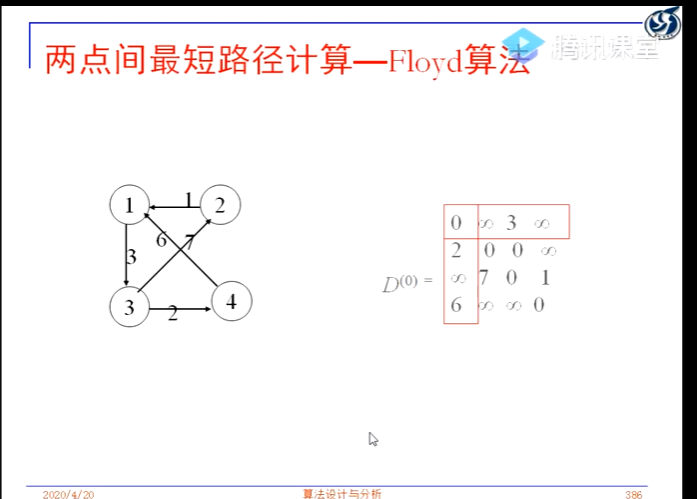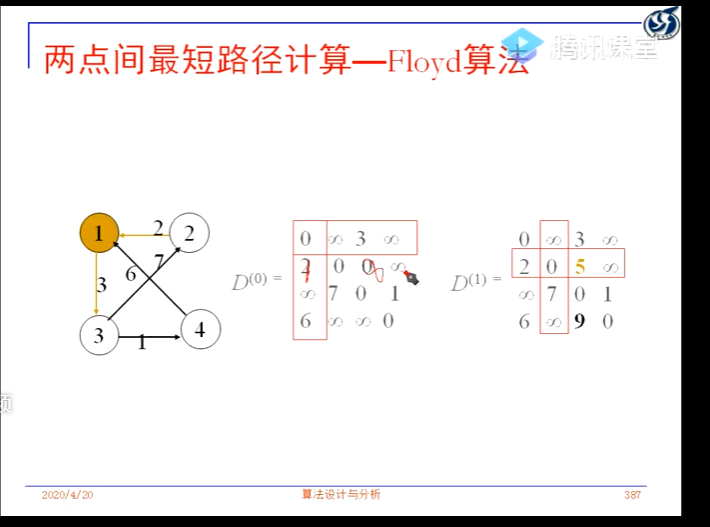伪代码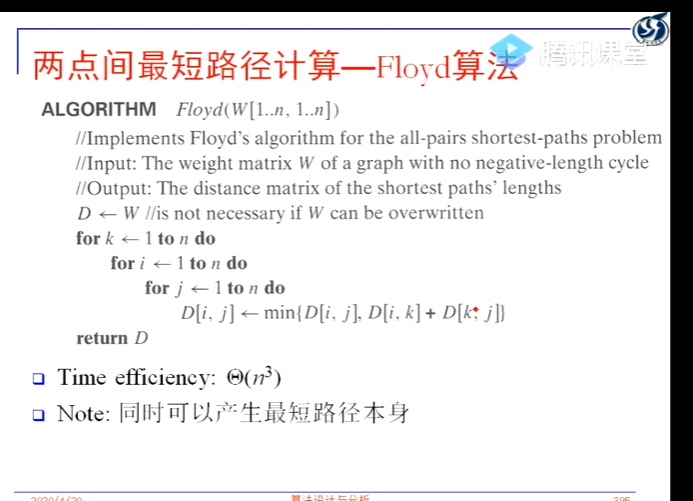完整代码
void floyd()
{
for (int k = 0; k < n; ++k)
{
for (int i = 0; i < n; ++i)
{
for (int j = 0; j < n; ++j)
{
//在当前i到j经过k点的路径与直连的路径中选最短
matrix[i][j] = min(matrix[i][j], matrix[i][k] + matrix[k][j]);
}
}
}
}



展开全文• 一点有关任意两点最短路径的思考！！仅仅是个人拙见。
• Dijkstra算法是求源点到其它顶点的最短路径。怎样求任意个顶点之间最短路径
• 本文主要讲述最短路径算法，一个主要原因是网上的“基于Matlab实现的两点之间最短路径算法”存在各种实现错误，目前为止还没有找到一个完全正确的。所以，本人改正相关错误，上传个正确的版本，即：采用Matlab实现的...
• 通过输入，能够实现找到最短路径。源代码能运行，简单易懂
• Dijkstra(迪杰斯特拉)算法是典型的单源最短路径算法，用于计算一个节点到其他所有节点的最短路径。主要特点是以起始为中心向外层层扩展，直到扩展到终点为止。Dijkstra算法是很有代表性的最短路径算法，在很多专业...
• /* *求有向网中的任意两点的最短路径 *弗洛伊德算法的核心是 运用一个path二维数组 和一个A...*运用三层循环 （算法的核心） 计算任意两点之间最短路径长度 将经过的节点的下标存储在path数组中去 *递增的思想 *创...C语言数据结构
• 其思路是将两点间距离分为过（指定的）第三点或是不过，然后取它们的最小值，如此循环就可以得到两点之间真正的最小值。 void floyd() { for (int k = 0; k < n; ++k) { for (int i = 0; i < n; ++i...
• 1 先求出原点离中间之间最短路径，然后，基于已经求出的最短路径，进一步求出它们之间最短路径。 转载于:https://www.cnblogs.com/wwwfj/p/3350166.html
• MAX——定义两点之间若无路径赋予的最大值 变量： DIST[N]——存储已经搜寻到的最短路径 Is[N]——存储节点是否被遍历的状态 Path[N]——图之间的路径矩阵 Road[N]——存储最短路径时该节点的上一节点 算法...Dijkstra算法
• // 保存start到其他各点最短路径的字符串表示 for (int i = 0; i ; i++){ path[i] = new String(point[start] + pathTip + point[i]); } int[] visited = new int[n]; // 标记当前该顶点的最短路径...
• #include <bits/stdc++.h> using namespace std; const int maxn = 100; const int inf = 0x3f3f3f3f; int n, m, s, d; int graph[maxn][maxn]; int dis[maxn][maxn]; void floyd() ... dis[i][j]算法 数据结构
• 防止相异的两点距离为0的情况出现  array[j][i]  =  array[i][j];  }  array[i][i]  =   0 ;  }   return  array;  }   // 注意：k 的循环要在最外层   static   int [][] shortestpath() ... {   ...string class 测试
• A*（A-Star)算法是一种静态路网中求解最短路最...　其中 f(n) 是从初始经由节点n到目标的估价函数， 　g(n) 是在状态空间中从初始节点到n节点的实际代价，（即N到起始位置的） 　h(n) 是从n到目标节点最...
• importjava.util.Scanner;//最短路径求解public classDistMin {static classGraphMatrix{static final int MaxNum=20;char[] Vertex=new char[MaxNum]; //保存顶点信息(序号或字母)int GType; //图的类型(0：...
• 给定一个乡镇网络图，求取个乡镇的最短路径，并输出路径，以及路径距离的大小。
• 需求动态查询两点之间最短路径(起点固定)；查询的路径高亮显示；Cesium 对生成的路径进行小车移动展示。技术实现路线动态查询两点之间最短路径 -> Postgresql 中的 PgRouting 实现；查询的路径高亮显示 ->...
• 注意：这里需要求解的最短路径指的是个城市之间的最短距离，而不是所有城市之间最短总距离。1.最短路径算法//最短路径算法static void distMin(GraphMatrix GM,int vend){ //vend为结束int[] weight=new in...
• 两点顶点之间最短路径问题 基于matlab的dijkstra算法 前言 看了很多篇介绍dijkstra算法的博客，没发现对于程序来说很多的地方都有问题。 一、函数代码 代码如下（示例）： function [ mydistance,mypath] = ...dijkstra 算法
• 注意：这里需要求解的最短路径指的是个城市之间的最短距离，而不是所有城市之间最短总距离。 1.最短路径算法 //最短路径算法 static void distMin(GraphMatrix GM,int vend){ //vend为结束......